Multi-Crystal Interferometry with Intensity Correlation

1. Why multi-crystal interferometer? And it's difficulty.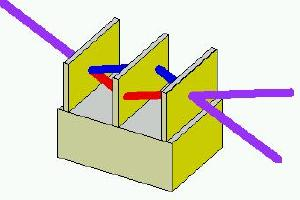Monolithic Laue-Laue-Laue Interferometer
In general interferometers require a stability of wavelength. This means X-ray interferometers need Angstrom stability. It is the strigent requirement why most researchers have made X-ray interferometers in a monolithic scheme.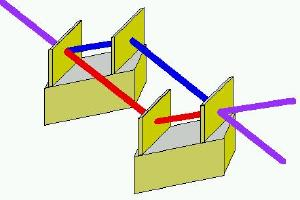Skew-symmetric triple Laue bicrystal Interferometer
Interferometers made from separate crystals have many possibilities.
1. One can scan only one component of the interferometer, such as the analyzer for lattice constant measurements.
2. One can obtain wider interference area for phase contrast imaging, than that limited by the size of Si ingot in case of monolithic interferometers.
3. One can get larger separation in the interferometer, where variable optics may be inserted.

But the strigent requirement of Angstrom stability and resolution.

2. Visibility and Intensity Correlation.

Suppose the output intensity, I, from the interferometer is exppressed as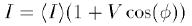,
where <I> is the average intensity, V is the visibility of the intensity oscillation, and phi is the phase variable. Its auto correlation is given by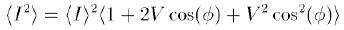.
Since phi is related to the angstrom scale, phi scans over wide range during measurements if special care was not take to stabilize the interferometer. Thus averaging by phi, one get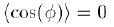, and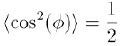.
Now measuring intensity correlation (auto correlation), the visibility of the oscillation can be decided by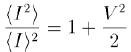.

3. Experimental Verification of Theory.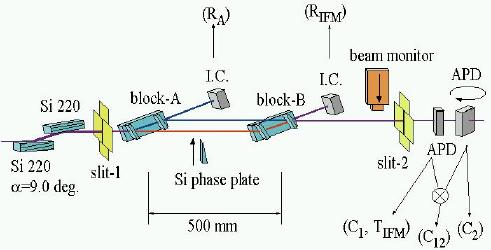Left figure is a schematic setup of the experiment. First two crystals were beam expander. Next two crystals consited the skew-symmetric LLL bicrystal interferometer. Each block was mounted on independent precision goniometer, and their separation was 500 mm.

The experminetal setup @ BL29XUL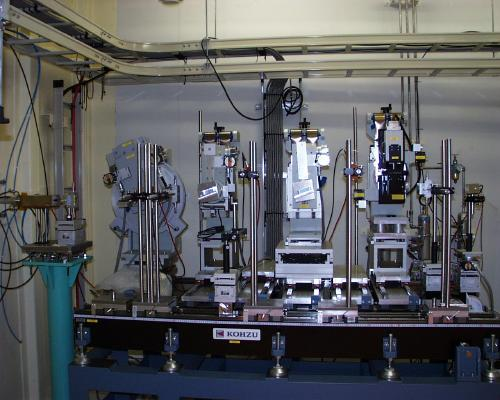4. Result and Discussion
to be written